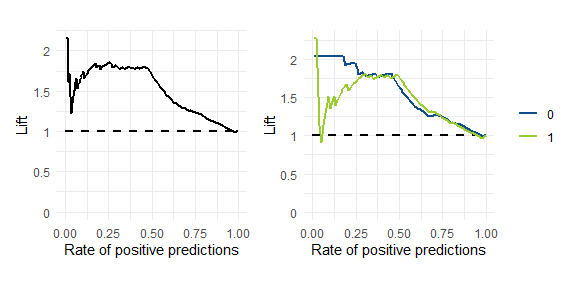# ezplot

## Overview

ezplot provides high-level wrapper functions for common chart types with reduced typing and easy faceting. e.g.:

• `line_plot()`
• `area_plot()`
• `bar_plot()`
• `tile_plot()`
• `waterfall_plot()`
• `side_plot()`
• `secondary_plot()`

## Installation

You can install the released version of ezplot from CRAN with:

``install.packages("ezplot")``

And the development version from GitHub with:

``````# install.packages("devtools")
devtools::install_github("wkostelecki/ezplot")``````

## Usage

``````library(ezplot)
library(tsibble)
library(tsibbledata)
suppressPackageStartupMessages(library(dplyr))
suppressPackageStartupMessages(library(lubridate))
suppressPackageStartupMessages(library(ggplot2))
library(patchwork)
suppressPackageStartupMessages(library(ROCR, warn.conflicts = FALSE))``````

### line_plot

Weekly aggregation:

``line_plot(ansett, x = "Week", y = "Passengers")``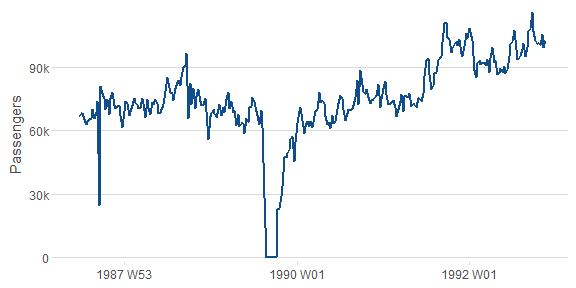Add grouping:

``line_plot(ansett, x = "Week", y = "Passengers", group = "Class")``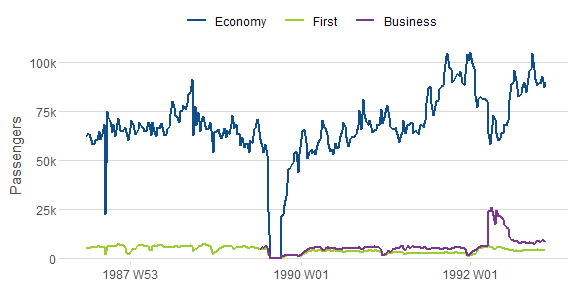Add faceting:

``````line_plot(ansett, x = "Week", y = "Passengers",
group = "Class", facet_x = "Airports",
facet_scales = "free_y") +
theme(axis.text.x = element_text(angle = 90, vjust = 0.38, hjust = 1))``````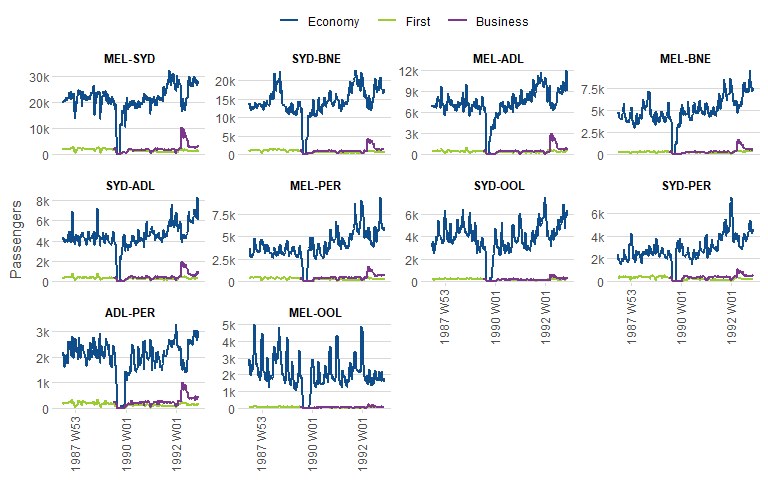Plot YOY comparisons:

``````line_plot(gafa_stock, "Date", c("Closing Stock Price" = "Close"),
facet_y = "Symbol",
facet_scales = "free_y",
yoy = TRUE,
labels = function(x) ez_labels(x, prepend = "\$"))``````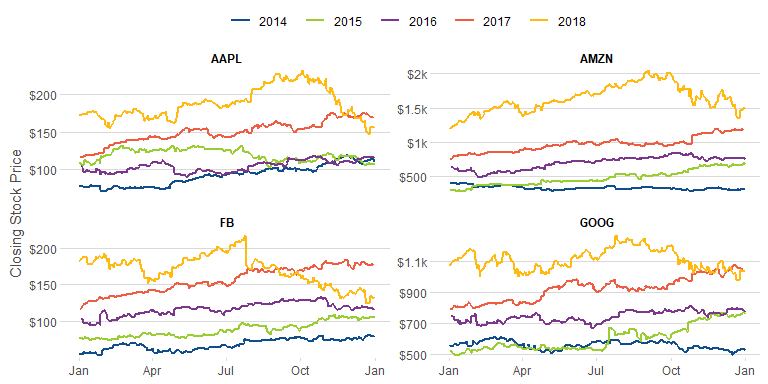Plot multiple numeric columns:

``````line_plot(hh_budget,
"Year",
c("DI", "Expenditure", "Savings"),
facet_x = "Country") +
theme(panel.spacing.x = unit(1, "lines")) +
ylab(NULL)``````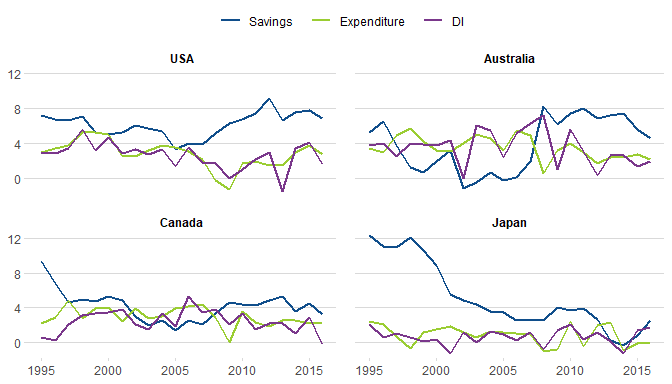### area_plot

Weekly aggregation:

``area_plot(ansett, x = "as.Date(Week)", y = "Passengers")``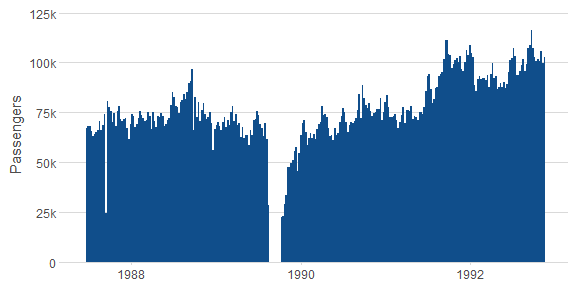Add grouping:

``````area_plot(ansett, x = "as.Date(Week)",
y = c("Weekly Passengers" = "Passengers"),
"Class")``````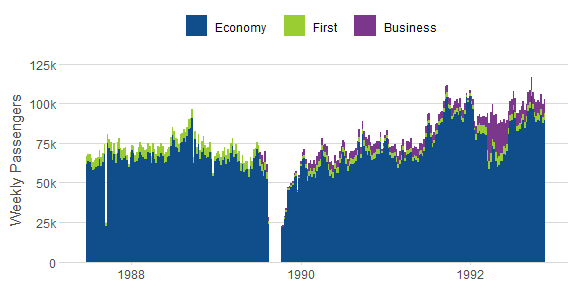Add faceting:

``````area_plot(ansett,
"year(Week) + (month(Week) - 1) / 12",
y = c("Monthly Passengers" = "Passengers"),
group = "substr(Airports, 5, 7)",
facet_x = "substr(Airports, 1, 3)", facet_y = "Class",
facet_scales = "free_y") +
theme(axis.text.x = element_text(angle = 90, vjust = 0.38, hjust = 1))``````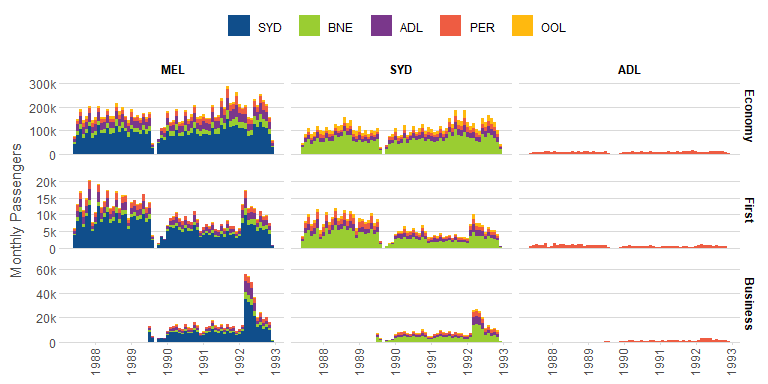### bar_plot

Yearly aggregation:

``````bar_plot(subset(aus_retail, year(Month) >= 2010),
x = "year(Month)",
y = "Turnover")``````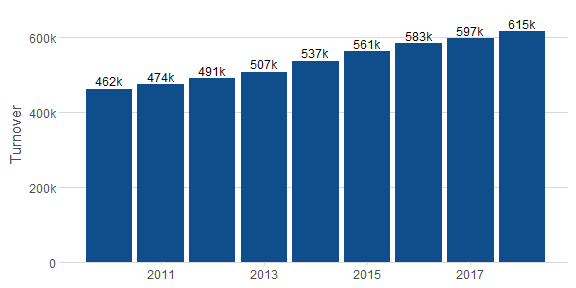With grouping:

``````bar_plot(subset(aus_retail, year(Month) >= 2010),
x = "year(Month)",
y = "Turnover",
group = "State")``````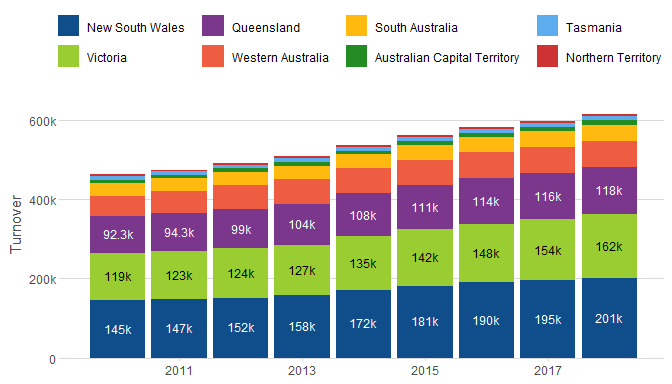Share of turnover:

``````bar_plot(subset(aus_retail, year(Month) >= 2010),
x = "year(Month)",
y = "Turnover",
group = "State",
position = "fill")``````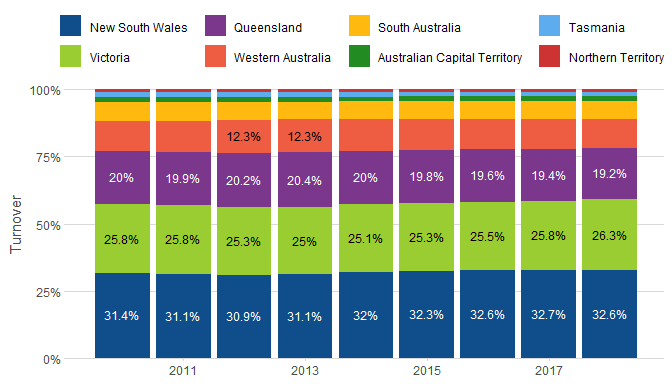### tile_plot

``````nyc_bikes %>%
mutate(duration = as.numeric(stop_time - start_time)) %>%
filter(between(duration, 0, 16)) %>%
tile_plot(c("Trip Start (Hour of Day)" = "lubridate::hour(start_time) + 0.5"),
c("Ride Duration (min)" = "duration - duration %% 2 + 1"))``````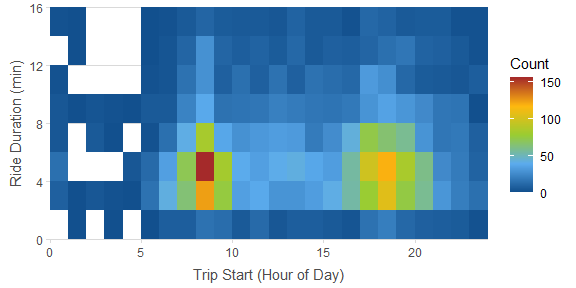``````tile_plot(vic_elec,
c("Temperature (C)" = "round(Temperature)"),
c("Half-Hourly Demand (MW)" = "round(Demand, -2)"),
labels_y = ez_labels, facet_x = "year(Time)")``````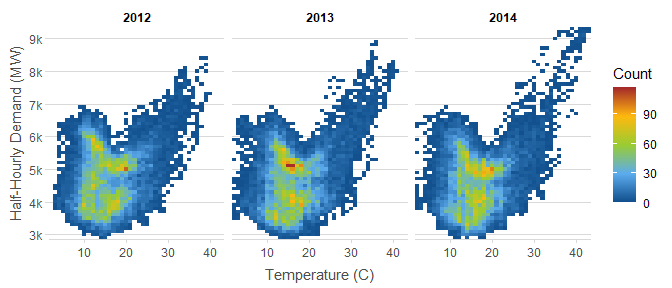### waterfall_plot

``````waterfall_plot(aus_retail,
"lubridate::year(Month)",
"Turnover",
"sub(' Territory', '\nTerritory', State)",
rotate_xlabel = TRUE)``````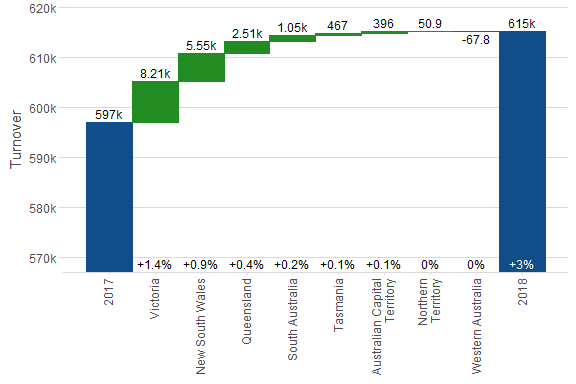### side_plot

``````side_plot(PBS,
"paste(Concession, Type, sep = ' - ')",
c("Scripts", "Cost", "Average Cost" = "~ Cost / Scripts"))``````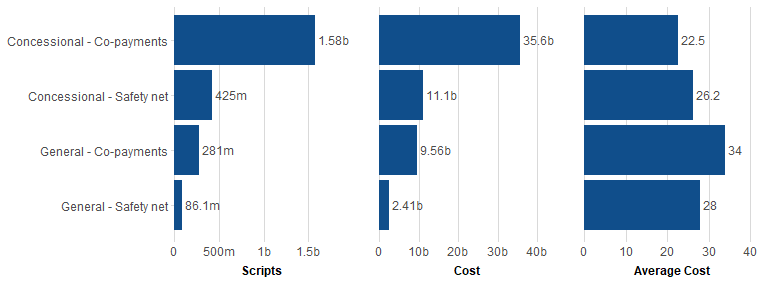### secondary_plot

Plot with secondary y-axis.

``````secondary_plot(pelt, "Year",
c("Hare Population" = "Hare"), c("Lynx Population" = "Lynx"),
ylim1 = c(0, 160e3),
ylim2 = c(0, 80e3))``````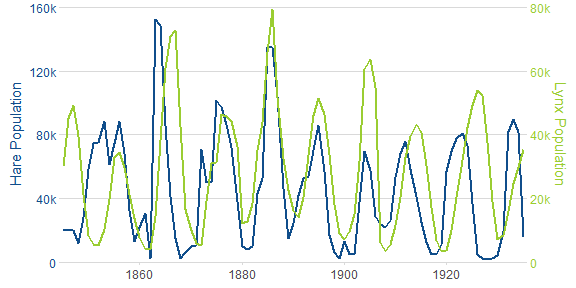### density_plot

``````nyc_bikes %>%
mutate(duration = as.numeric(stop_time - start_time)) %>%
density_plot(c("time of day" = "as.numeric(start_time) %% 86400 / 60 / 60"),
group = "ifelse(wday(start_time) %in% c(1, 7), 'week end', 'week day')")``````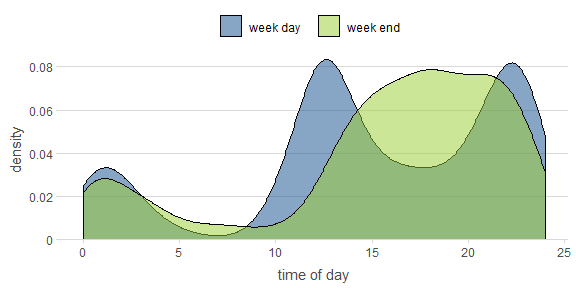### histogram_plot

``````nyc_bikes %>%
mutate(duration = as.numeric(stop_time - start_time)) %>%
histogram_plot(c("time of day" = "as.numeric(start_time) %% 86400 / 60 / 60"),
"density",
group = "ifelse(wday(start_time) %in% c(1, 7), 'week end', 'week day')",
position = "identity",
bins = 48)``````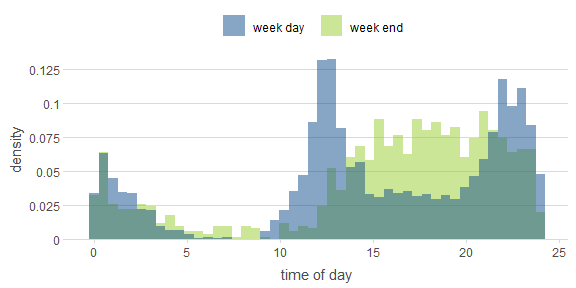### Classification data

``````data(ROCR.simple)
df = data.frame(pred = ROCR.simple\$predictions,
lab = ROCR.simple\$labels)
set.seed(4)``````

### roc_plot

``````set.seed(4)
roc_plot(df, "pred", "lab") +
roc_plot(df, "pred", "lab", group = "sample(c(0, 1), n(), replace = TRUE)")``````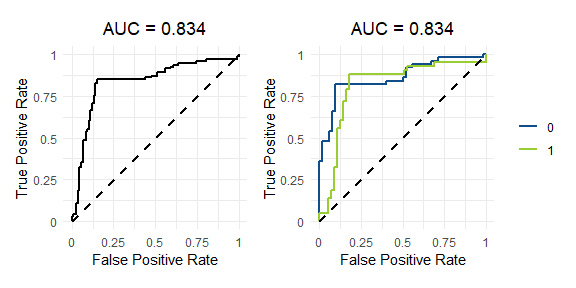### pr_plot

Precision-Recall plot

``````set.seed(4)
pr_plot(df, "pred", "lab") +
pr_plot(df, "pred", "lab", group = "sample(c(0, 1), n(), replace = TRUE)")``````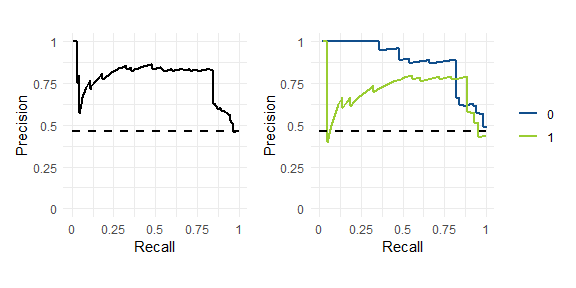### lift_plot

``````set.seed(4)
performance_plot(df, "pred", "lab", x = "rpp", y = "lift") +
performance_plot(df, "pred", "lab", group = "sample(c(0, 1), n(), replace = TRUE)", x = "rpp", y = "lift")``````